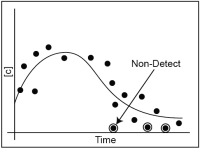## 5.7 Managing Nondetects in Statistical AnalysesEnvironmental statistics is constrained by a practical reality of laboratory analysis: it is technically impossible for a laboratory analysis to confirm the complete absence of a chemical or compound of interest. Instead, a chemical may be present at some unknown concentration below the low end of the concentration rangeThe difference between the largest value and smallest value in a dataset (NIST/SEMATECH 2012). that the analysis is able to detect. Since the true level is unknown, laboratories report the nonzero value representing the lowest concentration that can be reliably detected for the given analytical method. This alternate value is often used in environmental statistical applications, even though the true value can only be narrowed to a range of possible concentrations (for example, from zero up to the reporting limit).

### 5.7.1 Definition of Detection Limits

In environmental testing, a detection limit is the concentration that is statistically greater than the concentration of a method blank with a high level of confidence (typically, 99%), or the lowest level of a given chemical that can be positively identified when using a particular analytical method. Signal intensity below the detection limit cannot be reliably distinguished from a method blank or “baseline noise.” Therefore, an analyte is confidently reported as present in an environmental sample only when the measured concentration is greater than the detection limit.

Statisticians refer to any threshold at which a nondetect is reported as a “censoring limit.” Nondetects are sometimes referred to as censored values. Censoring limits affect how one should manage data. For instance, reporting nondetectsLaboratory analytical result known only to be below the method detection limit (MDL), or reporting limit (RL); see "censored data" (Unified Guidance). to larger censoring limits (higher detection limits) than needed tends to adversely impact data quality and increase data uncertainty. Unfortunately, different environmental testing laboratories use different types of censoring limits and reporting conventions for nondetects. No standard industry practice exists for establishing censoring limits.

### 5.7.2 Managing Nondetects

Despite considerable research in recent years on handling nondetects, regulatory agencies have published no comprehensive guidance on the recommended approach to use in a particular situation. As a result, approaches to handling nondetects in groundwater projects vary widely.

The following are the general strategies for handling nondetects:

1. Use statistical approaches specifically designed to accommodate nondetects, such as the Tarone-Ware two-sample alternative to the t-test.
2. Use a rank-based, nonparametricStatistical test that does not depend on knowledge of the distribution of the sampled population (Unified Guidance). test, such as the Mann-Kendall trend test.
3. Use a censored estimation technique to estimate sample statistics, such as the Kaplan-Meier method for calculating an upper confidence limit on the mean.
4. Impute an estimated value for each nondetect prior to further statistical analysis.

The most commonly used methods are described in the sections below.

### 5.7.6 Kaplan-Meier Method

The Kaplan-Meier method is a nonparametric technique for calculating the (cumulative) probability distribution and for estimating means, sums, and variances with censored data. Originally, the Kaplan-Meier approach was developed for right-censored survival data. More recently, the method was reformulated for left-censored environmental measurements (e.g., nondetects). USEPA’s Unified Guidance also recommends the Kaplan-Meier method for use as an intermediate step in calculating parametric prediction limits, control chartsGraphical plots of compliance measurements over time; alternative to prediction limits (Unified Guidance)., and confidence limits for censored data sets. In this latter application, the Kaplan-Meier estimate of the mean and variance is substituted for the sample mean and variance in the appropriate parametric formula.

### 5.7.7 Robust Regression on Order Statistics

Robust regression on order statistics (ROS) is a semi-parametric method that can be used to estimate means and other statistics with censored data. Unlike Kaplan-Meier, ROS internally assumes that the underlying population is approximately normal or lognormalA dataset that is not normally distributed (symmetric bell-shaped curve) but that can be transformed using a natural logarithm so that the data set can be evaluated using a normal-theory test (Unified Guidance).. However, the assumption is directly applied to only the censored measurements and not to the full data set (hence the term ‘semi-parametric’). In particular, ROS plots the detected values on a probability plot (with a regular or log-transformed axis) and calculates a linear regression line in order to approximate the parameters of the underlying (assumed) distribution. This fitted distribution is then utilized to generate imputed estimates for each of the censored measurements, which are then combined with the known (detected) values to summary statistics of interest (e.g., mean, variance). The method is labeled ‘robust’ because the detected measurements are used ‘as is’ to make estimates, rather than simply using the fitted distributional parameters from the probability plot.

### 5.7.8 Maximum Likelihood Estimation (Including Cohen's Method)

Maximum likelihood estimation (MLE) is a parametric, model-based method that can be used to estimate means and other summary statistics with censored data. In this approach, you must know or assess what distribution (such as normal or lognormal) will best model the data set. The model parameters for that distribution (mean and variance) are then estimated by maximizing the likelihood of the observed values, while simultaneously treating each nondetect as an inequality. Once the model parameters are determined, other statistics can be estimated from the model.

Cohen’s method (Chapter 15.5.1, Unified Guidance) is a simplified application of the MLE approach, where the underlying model is assumed to be normal (or transformed to normality) and the data contain but a single reporting limit, with all detected values larger than the nondetects.

Publication Date: December 2013

Permission is granted to refer to or quote from this publication with the customary acknowledgment of the source (see suggested citation and disclaimer).

This web site is owned by ITRC.

1250 H Street, NW • Suite 850 • Washington, DC 20005

(202) 266-4933 • Email: [email protected]

ITRC is sponsored by the Environmental Council of the States.